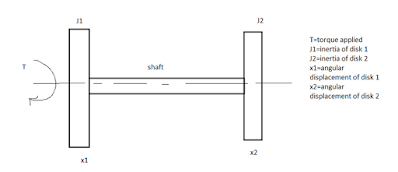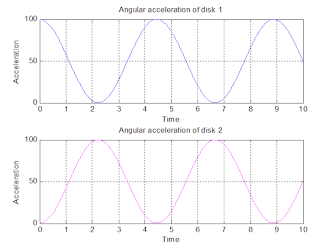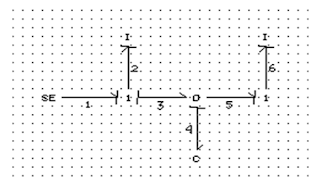## Welcome to the World of Modelling and Simulation

### What is Modelling?

This blog is all about system dynamics modelling and simulation applied in the engineering field, especially mechanical, electrical, and ...### Bond Graph Modelling, A Quick Learning: Part 5

Part 4 showed how bond graph represents the system differential equations; in this section, another basic example is highlighted.
The following figure 1 represents pretty simple model where two circular disks of inertia J1 and J2 are mentioned. Torque (T) is applied to the disk 1. The shaft has its own stiffness which is K.Fig. 1: Showing a very simple model where two disks are connected by a shaft and torque is applied to one of them.

Solutions of the Equations:

For solving the state equations to represent the system dynamics, we are going to use the bond graph method.
Parameter Values:
J1=100; J2=100; K=100; T=10000 (all values are considered unit less for simplicity)Fig. 2: Showing angular accelerations of disk 1 and 2.Fig. 3: Showing angular velocities of disk 1 and 2.Fig. 4: Showing angular displacements of disk 1 and 2.

So, from the above figures (2, 3 and 4), the system behaves quite well. It is also clear that both the disk's displacement and velocity increase with the increase of time.Fig. 5: Bond graph of the disk-shaft model.

I would like to explain the states and state equations from bond graph perspective. Bond graph depicts the picture of energy flow throughout the system and it considers the states which store energy which is eventually integrally causal element in the bond graph. Inertial (-I) and compliance (-C) elements are the energy storage elements. Bond graphs “bookkeep” energy and the instantaneous energy of the system is indicated by the energy variables (p’s on –I and q’s on ¬C). The dissipative elements (¬R) are not considered as state variable in bond graph formulation because the energy storage elements are independent if these can accept integral causality. Therefore, the state variables are the p (momentum)-variables on I- elements and the q- (displacement) variables on C-elements that are in integral causality.
From the above bond graph of our considered system (fig. 5), two inertial elements (disks 1 and 2) which has inertias I2, I6 and the state variables are denoted by p2 and p6. And, shaft is considered as compliant element which has compliance C4 is also treated as the states (q4).

The following equations are produced by CAMP-G.

e1=SE1;                  f2=P2/I2;
e4=Q4/C4;            f3=f2;
e5=e4;                    f6=P6/I6;
e6=e5;                    dP6=e6;
f1=f2;                      e3=e4;
f5=f6;                      e2=e1-e3;
f4=f3-f5;                dP2=e2;
dQ4=f4;

e1,e2,e3,e4,e5,e6 and f1,f2,f3,f4,f5,f6 are the efforts and flows throughout the system. These equations are sequential and eventually start from bond 1. From the equations,

dP2=e6= e1-e3= SE1- Q4/C4 -------- (1)

dP6=e6= e5=e4= Q4/C4--------- (2)

dQ4=f4= f3-f5= f2-f6= P2/I2- P6/I6 -------- (3)

Three differential equations are highlighted which are basically the state equations considered here. Here, three states are considered which differs from the state space method (which eventually considers four states). And, we see here the differential equations are expressed in details with the corresponding values substituted from the chronological equations (obtained by CAMP-G). Equations (1) and (2) are just equivalent with the two state space equations

And, if we notice carefully equation (3) represents basically the difference of another two state space equations.

Therefore, the notions are exactly same for both the cases. CAMP-G here basically considers all the necessary equations which are also obtained from state space model. The difference is CAMP-G considers three states (momentum of the two disks and shaft compliance) whereas state space method considered four states (angular displacements and velocities of the two disks). However, this does not pose any violation of the nature. We could essentially assume additional two states (positions) to find out the angular displacements of the disks. Again, these two additional states will create two differential equations just to calculate the positions of the disks.